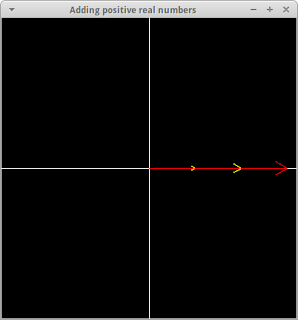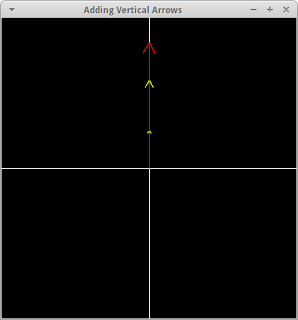# Learning Clojure

## Sunday, October 27, 2013

### Feynman' Arrows: OK, so What are the Complex Numbers?

```;; Feynman's Arrows II : OK, so what are the complex numbers?
;; requires [simple-plotter "0.1.2"]

;;;;;;;;;;;;;;;;;;;;;;;;;;;;;;;;;;;;;;;;;;;;;;;;;;;;;;;;;;;;;;;;;;;;;;;;;;;;;
;; Here's some code from the previous post

;; use pomegranate to get the library, if it's not already on your classpath
(require 'cemerick.pomegranate)
:coordinates '[[simple-plotter "0.1.2"]]
:repositories {"clojars" "http://clojars.org/repo"})

(use 'simple-plotter)

;; Make blackboards to draw arrows on
(defn make-blackboard [title size]
(create-window title 400 400 white black (- size) size (- size) size)
(axes)
(ink yellow))

;; Draw an arrow shape from (a,b) to (c,d)
(defn draw-offset-arrow [[a b][c d]]

;; Draw one of our arrows, which always have their tails at 0
(defn draw-arrow [[a b]] (draw-offset-arrow [0 0] [a b]))

;; Here's everything we know about the arrows so far:
[(+ a c) (+ b d)])

(defn multiply-arrows[[a b][c d]]
[(- (* a c) (* d b)) (+ (* a d) (* c b))])

;; That's easier to read in the standard prefix notation
;; (a,b)+(c,d) -> (a+b, c+d)

;; The addition rule says: treat arrows as if they represented vector displacements, and add them nose to tail
;; The multiplication rule says: treat arrows as if they were zooms and rotations, and use one to zoom and rotate the other.

;; We also had a few favourite arrows that we'd played with:
(def arrow1 [3,4])
(def arrow2 [4,-3])
(def arrow3 [1,1/10])

;;;;;;;;;;;;;;;;;;;;;;;;;;;;;;;;;;;;;;;;;;;;;;;;;;;;;;;;;;;;;;;;;;;;;;;;;;;;

(do (make-blackboard "Favourite Arrows" 6)
(doseq [i [arrow1 arrow2 arrow3]] (draw-arrow i)))```

```;;;;;;;;;;;;;;;;;;;;;;;;;;;;;;;;;;;;;;;;;;;;;;;;;;;;;;;;;;;;;;;;;;;;;;;;;;;;

;; The first thing I want you to notice is that there's a subset of
;; the arrows that works exactly like the numbers that we all got
;; comfortable with in primary school. They're the ones where the
;; arrows point due east or west.

(add-arrows [10 0] [5 0]) ;-> [15 0]
;; (10,0) + (5,0) -> (15,0) is like 10 + 5 -> 15

(do (make-blackboard "Adding positive real numbers" 16)
(draw-arrow [5 0])
(draw-arrow [10 0])
(ink red)
(draw-arrow (add-arrows [10 0] [5 0])))(multiply-arrows [4 0] [3 0]) ;-> [12 0]
;; (4,0) * (3,0) -> (12,0) is like 4 * 3 -> 12

(do (make-blackboard "Multiplying positive real numbers" 16)
(draw-arrow [4 0])
(draw-arrow [3 0])
(ink red)
(draw-arrow (multiply-arrows [4 0] [3 0])))

(multiply-arrows [-1 0] [-2 0]) ;-> [2 0]
;; (-1,0) * (-2,0) -> (2,0) is like   -1 * -2 -> 2

(do (make-blackboard "Multiplying negative real numbers" 3)
(draw-arrow [-1 0])
(draw-arrow [-2 0])
(ink red)
(draw-arrow (multiply-arrows [-1 0] [-2 0])));; You probably learned 'minus times minus is plus' as an arbitrary
;; rule, but it's obvious if you think of -1 as meaning 'leave it the
;; same size but turn it 180 degrees.'

;; We say that the 'real numbers' are 'embedded' in the arrows.

;; What we mean is that the horizontal arrows behave just like the
;; real numbers, and so in any place where we were going to use real
;; numbers we can just use horizontal arrows instead and everything
;; will work out exactly the same.

;; And so since it doesn't matter whether we think about (3,0) or 3,
;; we'll usually just forget about the difference, and sometimes write (3,0),
;; and sometimes write 3, depending on convenience.

;; So now I can say -3 * 4 -> -12, and that's a statement about arrows!

;; There's another subset of the arrows, that point due north and due south.

;; Under addition, they're just like the real numbers too.

;; (0, 7)+ (0, 3) -> (0, 10)

(add-arrows [0 7] [0 3]) ;-> [0 10]

(do (make-blackboard "Adding Vertical Arrows" 12)
(draw-arrow [0 7])
(draw-arrow [0 3])
(ink red)
(draw-arrow (add-arrows [0 7] [0 3])));; But when you multiply them, they end up turning each other into horizontal arrows
;; (literally 'turning')

;; (0, 2) * (0, 3) -> (0x0-2x2, 0x3+2x0) = (-6, 0)

(multiply-arrows [0 2] [0 3]) ;-> [-6 0]

(do (make-blackboard "Multiplying Vertical Arrows" 12)
(draw-arrow [0 2])
(draw-arrow [0 3])
(ink red)
(draw-arrow (multiply-arrows [0 2] [0 3])));; You can see why pretty easily.

;; (0, 10) represents 'turn 90 degrees clockwise and magnify by 10',
;; and (0, 4) means 'turn 90 degrees clockwise and magnify by 4'

;; And the product (-40, 0) means turn 180 degrees and multiply by 40.

;; In this view, (-1, 0), or just -1 means 'turn 180 degrees (no zooming!)'

;; And the (0, 1) * (0, 1) -> (-1, 0) is just the fact 'if you turn 90
;; degrees anticlockwise, and then you turn another 90
;; degrees anticlockwise, then that's the same as if you'd
;; turned 180 degrees'.

;; So as long as we're talking about arrows, the thing we've called
;; -1, or the pair (-1,0), or the arrow length 1 that points east, or
;; the idea of turning through 180 degrees, does have a 'square root'.

;; There is a thing, the arrow length 1 that points straight north, or
;; the pair (0,1), or the idea of turning 90 degrees, that if you
;; multiply it by itself you get -1.

;; That's important, but it's also trivial.

;; Two quarter-turns clockwise make a 180 turn.

(multiply-arrows [0,1] [0,1]) ;-> [-1 0]

;; Two quarter-turns make a half-turn
(do (make-blackboard "Something Whose Square is (-1,0)" 2)
(draw-arrow [0,1])
(draw-arrow [0,1])
(ink red)
(draw-arrow (multiply-arrows [0,1] [0,1]))))
```

```;; We call that upwards pointing, length 1 arrow i, for historical reasons.
(do (make-blackboard "The Mysterious and Magical i" 2)
(draw-arrow [0,1])))
```

```;; And those vertical numbers, again, for historical reasons, are
;; called Imaginary Numbers.

;; Why on earth would anyone call sideways-pointing arrows 'real' and
;; upwards-pointing arrows 'imaginary'?

;; Well, it's hard to say exactly. The mathematicians of the 17th
;; century weren't thinking about rotations and scalings. They were
;; thinking about roots of equations.

;; Despite what's usually said, it never bothered them in the
;; slightest that x^2+1 didn't have a solution.  It was completely
;; obvious that it couldn't have, and that was fine.

;; What did worry them is that they had a formula for the cubic
;; equation, and when they used it to solve cubic equations that
;; really obviously did have three roots, like x^3=x, which is true
;; when x is -1, and when x is 0, and when x is 1, their formula kept
;; insisting that they do weird things, like taking the cube root of
;; the square root of -1.

;; And they had no idea what that meant, but some brave ones just ran
;; with the idea and found that if you followed the absurdity through
;; far enough then at the end the formula would give you the right

;; But they were, quite reasonably, very suspicious of the whole
;; procedure, and they called these weird things 'fictitious', or
;; 'imaginary' numbers.

;; I'm not sure why it was so important to solve cubic equations in
;; the 17th century. But they used to do it a lot, apparently. And
;; often the formula was the only way to get the answers.

;; And of course because they didn't really know what they were doing,
;; they got very confused and kept making mistakes, but nevertheless,
;; they did keep getting the right answers to their problems.

;; Eventually the breakthrough came in 1799, when a Danish
;; cartographer, Caspar Wessel, published 'On the Analytical
;; Representation of Direction', where he thought about using numbers
;; to represent directions and distances.

;; In this ground-breaking paper, Wessel both invented the idea of the
;; vector, and realized that the vectors that he was using to
;; represent directions and rotations and scalings were the same thing
;; as the 'imaginary numbers' that had been scaring and confusing
;; people for nearly two hundred years.

;; Unfortunately, "Om directionens analytiske betegning", was
;; published in Danish by the Royal Danish Academy of Sciences and
;; Letters, and so no-one could understand it, and so it vanished
;; without trace.

;; Luckily, seven years later, a bookstore manager in Paris,
;; Jean-Robert Argand, made the same discovery, and immediately
;; polynomial equations as being about arrows, then it was blindingly
;; obvious what was going on.

;; Indeed Argand realised that the question
;; 'What are the roots of x^2 + 1 =0?'
;; is the same question as 'What do you have to do twice to turn round?'.

;; And he went quickly from that to showing that any such question has
;; an answer, which is called the Fundamental Theorem of Algebra.

;; And Argand published his idea in French, which is why today the
;; pictures of arrows that people use to reason about directions and
;; rotations and scalings are called 'Argand Diagrams'.

(do (make-blackboard "Wessel^h^h^h^h^h^h Argand Diagram" 10)
(dotimes [i 100] (draw-arrow [(- (rand-int 20) 10) (- (rand-int 20) 10)])));; Anyway, even though things that point upwards aren't really any
;; more imaginary than things that point sideways, the name Imaginary
;; Numbers has stuck, and it has the twin virtues of:

;; (a) Making the Complex Numbers look Really Cool and Mysterious,
;; adding to the Aura of Mathematics.

;; (b) Scaring and Confusing Scientists and Engineers, and indeed
;; anyone who needs to think about things that rotate and get bigger
;; or smaller. Mathematicians love doing this.

;; Engineers are practical people, and they use complex numbers all
;; the time when thinking about electricity and whether buildings will
;; fall over, and whether bridges will stay up, and eventually they
;; just accept that it's a really useful incomprehensible mystery and
;; get used to it. They say things like 'a capacitor is an imaginary
;; resistor', without even appearing to notice how strange that is.

;; But I think everyone else is puzzled and a bit frightened. I once
;; met a man with a PhD in Quantum Mechanics, who said that he
;; couldn't really believe that Quantum Mechanics was the true theory
;; of the world, because how could a thing that dealt with imaginary
;; quantities describe reality?

;; There is no need for any of this. Just Arrows. Just Rotations. Not
;; Scary. Children can do this.

;;;;;;;;;;;;;;;;;;;;;;;;;;;;;;;;;;;;;;;;;;;;;;;;;;;;;;;;;;;;;;;;;;;;;;;;

;; Anyway, if 1 is another name for (1,0), and i is another name for
;; (0,1), then notice that 5+3i is (5,0)+(3,0)*(0,1) -> (5,3)

;; So we have yet another way of describing our pairs:

(defn print-arrow [[a b]]
(str "the pair (" a "," b "), "
"also known as the complex number " a "+"b"i, "
"also known as the arrow " (cond (> a 0) (str a " north") (= a 0) "" :else (str (- a) "south"))
" and " (cond (> b 0) (str b " east") (= b 0) "" :else (str (- b) " west"))))

(print-arrow arrow1) ;-> "the pair (3,4), also known as the complex number 3+4i, also known as the arrow 3 north and 4 east"
(print-arrow arrow2) ;-> "the pair (4,-3), also known as the complex number 4+-3i, also known as the arrow 4 north and 3 west"
(print-arrow arrow3) ;-> "the pair (1,1/10), also known as the complex number 1+1/10i, also known as the arrow 1 north and 1/10 east"
(print-arrow [1/11 0.25]) ;-> "the pair (1/11,0.25), also known as the complex number 1/11+0.25i, also known as the arrow 1/11 north and 0.25 east"
```

#### 1 comment:

1.This is a brilliant example of literate programming in Clojure.